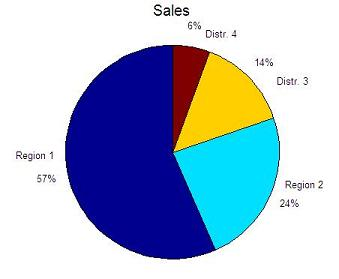# Pie Plots - figures in Matlab

pie(x)
draws pie plots of the data in the vector x. The values in x are normalized via x/sum(x) to determine the area of each slice of pie.

If sum(x) is less or equal to 1, the values in x directly specify the area of the pie slices.  Only a partial pie will be drawn if sum(x) is less than < 1.

The '%' sign indicates that there is a comment in that line and Matlab does not do anything with it. It is as if it were inexistent, and it exists only for explanatory purposes.

Example:

% Clears variables, command window, and closes all figures
clc; clear; close all

% These are the names of the slices
names = char('Region 1', 'Region 2', 'Distr. 3', 'Distr. 4');

% These are the numbers to be plotted
data = [1200, 500, 300, 120];
pie(data)

% gtext('string') displays the graph window, puts up a
% cross-hair, and waits for a mouse button or keyboard
% key to be pressed.
for i=1:4
gtext(names(i,:));
end
title('Sales', 'fontsize', 15)

The result is:From 'Pie Plots' to home

From 'Pie Plots' to 'Matlab Plots'